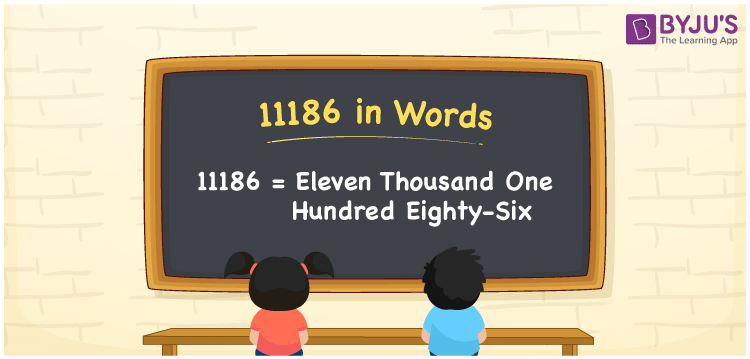# 11186 in Words

11186 in words can be written as Eleven Thousand One Hundred Eighty-six. The fundamental concepts in Mathematics like counting or count can be learnt efficiently here. If you buy a toy for Rs. 11186, then you can say that “I bought a toy for Eleven Thousand One Hundred Eighty-six Rupees”. To write numbers in words, the English alphabet is used. The numbers in words concept is explained here in a simple way to improve the conceptual knowledge of students. The 11186 can be read as “Eleven Thousand One Hundred Eighty-six” in English.

 11186 in words Eleven Thousand One Hundred Eighty-six Eleven Thousand One Hundred Eighty-six in Numbers 11186

## 11186 in English Words## How to Write 11186 in Words?

Students can learn about the expanded form and the place value chart of 11186. Five digits are present in the number 11186. With the help of the place value chart given below, students will be able to understand the concepts with ease.

 Ten Thousands Thousands Hundreds Tens Ones 1 1 1 8 6

11186 can be written in expanded form as:

1 x Ten Thousand + 1 x Thousand + 1 × Hundred + 8 × Ten + 6 × One

= 1 x 10000 + 1 x 1000 + 1 × 100 + 8 × 10 + 6 × 1

= 10000 + 1000 + 100 + 80 + 6

= 11186

= Eleven Thousand One Hundred Eighty-six

Hence, 11186 in words is written as Eleven Thousand One Hundred Eighty-six.

11186 is a natural number that precedes 11187 and succeeds 11185.

11186 in words – Eleven Thousand One Hundred Eighty-six

Is 11186 an odd number? – No

Is 11186 an even number? – Yes

Is 11186 a perfect square number? – No

Is 11186 a perfect cube number? – No

Is 11186 a prime number? – No

Is 11186 a composite number? – Yes

## Frequently Asked Questions on 11186 in Words

Q1

### How to write 11186 in words?

11186 can be written in words as “Eleven Thousand One Hundred Eighty-six”.
Q2

### How to write Eleven Thousand One Hundred Eighty-six in numbers?

Eleven Thousand One Hundred Eighty-six in numbers can be written as 11186.
Q3

### Is 11186 an odd or even number?

11186 is an even number as it is completely divisible by 2.